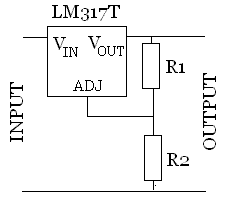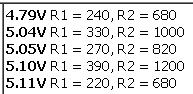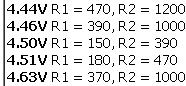# Using The LM317T To Regulate VoltageThe LM317T Voltage Regulator is an integrated circuit which is very useful in many renewable energyapplications. It can be used to regulate current – for example to regulate the current in a string of LEDs – or it can be used to provide a stable fixed voltage output.

* We have LM317T integrated circuits available here in the REUK Shop.

### Regulating Voltage with an LM317T

The voltage output from an LM317T is set simply using two resistors: R1 and R2.R1 is typically chosen to be a resistor of around 240 Ohm, and should be in the range of 100-1000 Ohms. The voltage across R1 is 1.25 volts – the reference voltage from the ADJ leg of the LM317T – and so a 1.25/240 = 5 milliamp current flows through R1. Using a 120 Ohm resistor for R1 for example would give the regulator a load of 10 milliamps which helps to give slightly better output voltage accuracy.

The value of R2 is then used to set the output voltage of the regulator according to the following equation:

 VOUT = 1.25 * ( 1 + R2/R1 )

which can be rearranged to give:

 R2 = R1 * ( (VOUT/1.25) -1 )

We have a handy LM317 Voltage Calculator which calculates R2 for any given value of R1 and desired output voltage. On the same page are two comprehensive tables (one grid, and one sorted list) presenting the output voltages given by different combinations of the most commonly found stock resistors.

### Example – Recharging a Mobile Phone from a 12V Battery Bank with an LM317T Regulator

If you decided to recharge your mobile phone battery directly from a 12 Volt (nominally) battery bankyou would typically need a voltage output of around 5 volts, and an output current of around 500ma. The LM317T can handle average currents of up to 1.5 Amps so it will work well in this situation.

Assuming that a standard 240 Ohm resistor is used as R1 all we have left to do is calculate the value ofR2 with a target output voltage of 5V:

 R2 = 240 * ( (5/1.25) -1 ) = 720

Therefore since no-one manufactures 720 Ohm resistors, use the next larger available size – a 750 Ohm resistor – to give a 5.16V output from the regulator.

Looking at the REUK LM317 Voltage Calculator for combinations of R1 and R2 which give outputs of around 5V we see the following options with common stock resistors:…so, the best combination to go for would be R1 = 330R and R2 = 1K for a 5.04V output voltage for our phone charging.

### LM317T Voltage Regulation Accuracy

In all these calculations it is important to remember that typical resistors have error ranges of up to 5%, and that the reference voltage of the LM317T can vary from 1.2 to 1.3 Volts – another 5% of error. Therefore if you cannot afford for the output voltage to be as much as ±10% away from the required voltage – an adjustable trimming potentiometer should be used for all or part of R2 enabling you to adjust the value of R2 until the output voltage is the chosen value.

Continuing with the example from earlier about charging a mobile phone, +/- 10% of 5V gives a range of 4.5 to 5.5V. Many phones will not charge at all with a voltage of 4.5V, and 5.5V could lead to overheating, battery damage, or again failure to charge at all.

Rather than selecting fixed values for R1 and R2, it would be better to fix R1 and select make R2 up of the fixed resistor which gives an output around 4.5V and a potentiometer (variable resistor) with a value which when connected in series to the fixed resistor and paired with R1 gives an output of around 5.5V. The potentiometer can then be adjusted until the output (measured with a voltmeter or multimeter) is exactly 5.00V).According to the REUK LM317 Voltage Calculator, R1 = 180R and R2 = 470R gives an output of 4.51V. Keeping R1 fixed as 180R and using the calculator again, 5.5V will be given by a value of R2 of 684R. We therefore need a potentiometer of 684-470=214 Ohms to cover the 4.5-5.5V range.

100R, 220R, and 470R are common potentiometer values, so the 220R is the perfect choice giving a theoretical maximum output voltage from the regulator of just over 5.5V (though potentiometers typically have an error bound of as much as +/- 20% so we could get anything from 5.3-5.7V as the actual maximum output voltage).

(Here is a link to our somewhat outdated 2007 article on Making a Solar iPod Charger which shows how to couple a PV solar panel with a 5V LM317 voltage regulator and a female USB socket to charge an iPod.)